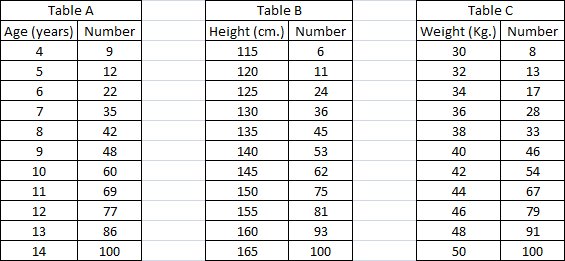### CAT 2003 Question Paper (Leaked) Question 23

Instructions

DIRECTIONS for the following three questions: Answer the questions on the basis of the information given below.Table A below provides data about ages of children in a school. For the age given in the first column, the second column gives the number of children not exceeding the age. For example, first entry indicates that there are 9 children aged 4 years or less. Tables B and C provide data on the heights and weights respectively of the same group of children in a similar format. Assuming that an older child is always taller and weighs more than a younger child, answer the following questions.

Question 23

# What is the number of children of age 9 years or less whose height does not exceed 135 cm?

Solution

Number of children of age 9 years or less are 48 and those whose height does not exceed 135 cm are 45 . 45 is lesser, hence answer is 45.

• All Quant CAT Formulas and shortcuts PDF
• 30+ CAT previous papers with solutions PDF